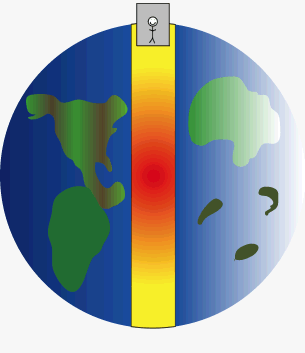Home » Posts tagged 'Isaac Newton'

# Tag Archives: Isaac Newton

## Gravity

We all know there is no such thing as zero gravity even at the very end of universe there is micro gravity according to formula for gravity…!
Means we are away from certain object so the gravity exist in vacuum so now my question is:-
Q- We all know that there is zero Gravity in center of earth core so if we place an object in center of earth the would Gravity formula apply on that object or that object is in complete Zero gravity…????

If we simply keep a body at the centre, it will remain there as it is attracted equally in all directions.A man in an elevator free falls through a planet to simulate how a gravity train could work. At both ends of the planet the train comes to a complete stop, but free falls through the middle (or wherever the tunnel is constructed) of the planet. (Photo credit: Wikipedia)

# GRAVITY## Gravity and Galileo

How Galileo gave acceleration due to gravity before Newton discovered gravity . exactly what happenNewton stated the Universal Law of gravitation and explained why objects fall to earth when dropped.

But objects were falling even before Newton explained it.

The concept of speed and velocity was known before the Law of Gravitation was formulated.## Some Physicists and their contributions

Physicists outside India

• Galileo – (formulated the basic law of falling bodies, constructed a telescope and discovered four moons revolving around Jupiter)

• Archimedes: Archimedes Principle and theory of floatation, Principle of levers)
• Albert Einstein: Theory of relativity, (Special and General)
• Isaac Newton: Laws of motion, The method of Calculus
• Michael Faraday: Electromagnetic induction, dynamo

Physicists from India## Refraction through a prism

For an equilateral prism , find two angle of incidence differing by 20 degrees showing same deviation of 40 degrees?

Use the formula

Here A = 60 degree (Since equilateral prism)

deviation is 40 degree

This gives

Therefore,

the values of angles of incidence are 60 degree and 40 degree## Newton’s Third Law of Motion and some doubts

Newton’s Third Law of Motion states that

“Every action has equal and opposite reaction”

In other words,

“Whenever a body exerts a force on another body, the second body exerts an equal and opposite force on the first body”“if a person is sitting on a chair both the chair and person exert force against it so we called that ” there is always an equal and opposite reaction ” but for example a elephant of mass 584 kg sit on a chair, the chair will broke.  So what we called for this . Please explain.

When an elephant “sits” on a chair, it breaks because it cannot give the “equal reaction” and  it “yields” to it.

Therefore, here the force is doing “work”.

More discussions are welcome## Doubt in Law of GravitationNewton's law of universal gravitation for two bodies. This law governs gravitational forces in the Earth. (Photo credit: Wikipedia)According to law of gravitation
F=g*(m1*m2)/r^2
If we bring two objects close to each other say our hands then the distance will be zero and the force of attraction will become infinite because r will we zero. Therefore F will become infinite but this is not the case.
When we touch our both palms together we can easily pull then away.

When we consider the distance, it is between the center of mass of the two bodies under consideration. When we bring our hands together the distance between their CM is not zero.

Other wise, the two particles under consideration must be point masses.

Then if you consider two atoms or two nuclei, the forces that come into play will be different if we decrease the distance between two atoms or nuclei. The attraction will turn into strong repulsion when the limits are exceeded.### Visitors So Far @ AskPhysics

• 2,166,689 hits

### Subscribe to Blog via EmailAsk Physics